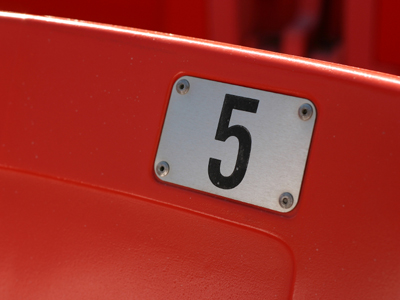The square root of 25 is 5.

# Irrational Numbers

This Math quiz is called 'Irrational Numbers' and it has been written by teachers to help you if you are studying the subject at middle school. Playing educational quizzes is a fabulous way to learn if you are in the 6th, 7th or 8th grade - aged 11 to 14.

It costs only \$12.50 per month to play this quiz and over 3,500 others that help you with your school work. You can subscribe on the page at Join Us

An irrational number is a number that cannot be fully written down into a simple whole number or a fraction. Rather it is written in decimal form but it has an infinite number of digits that extend to the right of the decimal point.

One well known irrational number is pi.

This is the circumference of a circle divided by its diameter. This number is the same for every circle. The number pi (written as π) is approximately 3.14159265358979323…. The ellipsis (…) shows that the number continues onward to infinity.

Most irrational numbers are found in square roots.

To square a number means to multiply the number by itself. For example, 5 x 5 is squaring the number “5”. This can also be stated as five squared which will then look like this “52” with the little exponent “2”. (5 x 5 = 25)

As the answer here is the number “25”, it is stated as the “square root of 25” is “5” because “5” multiplied by itself gave us “25”.

Square roots are either “perfect” meaning the whole number has a squared number that, when multiplied by itself will equal the number, i.e., 9 is a perfect number because 3 x 3 = 9, or they are “imperfect” which means there is no number when multiplied by itself will equal the square root. For example, the number 15 has no number that when multiplied by itself will give you the number 15. Therefore, 15 is an “imperfect root” and it will provide us with an “irrational” number.

The math symbol that represents a square root is: “√”. So using the example above it would read:

√25 = 5

Because there is no actual solution to finding a square root, the use of calculators is generally needed. In fact, for this quiz a calculator will be a must to find the irrational number. Although an irrational number can go to an infinite distance to the right of a decimal, for this quiz we will only go as far as four digits to the right.

1.
√17
3.9879
4.1231
4.0578
4.1369
The square root of 17 is 4.1231056. Cutting off with the fourth digit to the right of the decimal point we are left with 4.1231. Answer (b) is the correct answer
2.
√3
1.7320
1.7445
1.7493
1.7501
The square root of 3 is 1.7320508. Cutting off with the fourth digit to the right of the decimal point we are left with 1.7320. Answer (a) is the correct answer
3.
√123
10.9868
11.0905
11.0975
11.1543
The square root of 123 is 11.0905365. Cutting off with the fourth digit to the right of the decimal point we are left with 11.0905. Answer (b) is the correct answer
4.
√13
3.5691
3.5698
3.6055
3.6132
The square root of 13 is 3.6055513. Cutting off with the fourth digit to the right of the decimal point we are left with 3.6055. Answer (c) is the correct answer
5.
√29
5.3851
5.3794
5.3785
5.3697
The square root of 29 is 5.3851648. Cutting off with the fourth digit to the right of the decimal point we are left with 5.3851. Answer (a) is the correct answer
6.
√55
7.0987
7.3659
7.4161
7.5243
The square root of 55 is 7.4161985. Cutting off with the fourth digit to the right of the decimal point we are left with 7.4161. Answer (c) is the correct answer
7.
√21
4.5815
4.5825
4.5835
4.5845
The square root of 21 is 4.5825757. Cutting off with the fourth digit to the right of the decimal point we are left with 4.5825. Answer (b) is the correct answer
8.
√47
6.7496
6.7543
6.7579
6.8556
The square root of 47 is 6.8556546. Cutting off with the fourth digit to the right of the decimal point we are left with 6.8556. Answer (d) is the correct answer
9.
√33
5.7283
5.7297
5.7364
5.7445
The square root of 33 is 5.7445626. Cutting off with the fourth digit to the right of the decimal point we are left with 5.7445. Answer (d) is the correct answer
10.
√11
3.3212
3.5000
3.3166
3.3498
The square root of 11 is 3.3166248. Cutting off with the fourth digit to the right of the decimal point we are left with 3.3166. Answer (c) is the correct answer
Author:  Christine G. Broome